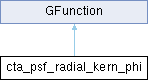GammaLib  1.7.0.dev

Kernel for Psf phi angle integration used for stacked analysis. More...

#include <GCTAResponse_helpers.hpp>## Public Member Functions

cta_psf_radial_kern_phi (const GModelSpatialRadial *model, const GEnergy &srcEng, const GTime &srcTime, const double &sin_fact, const double &cos_fact)

double eval (const double &phi)
Kernel for azimuthal radial model integration. More...Public Member Functions inherited from GFunction
GFunction (void)
Void constructor. More...

GFunction (const GFunction &function)
Copy constructor. More...

virtual ~GFunction (void)
Destructor. More...

GFunctionoperator= (const GFunction &function)
Assignment operator. More...

## Protected Attributes

GEnergy m_srcEng
True photon energy. More...

GTime m_srcTime
True photon arrival time. More...

double m_sin_fact
sin(delta)*sin(delta_mod) More...

double m_cos_fact
cos(delta)*cos(delta_mod) More...Protected Member Functions inherited from GFunction
void init_members (void)
Initialise class members. More...

void copy_members (const GFunction &function)
Copy class members. More...

void free_members (void)
Delete class members. More...

## Detailed Description

Kernel for Psf phi angle integration used for stacked analysis.

Definition at line 1178 of file GCTAResponse_helpers.hpp.

## Constructor & Destructor Documentation

 cta_psf_radial_kern_phi::cta_psf_radial_kern_phi ( const GModelSpatialRadial * model, const GEnergy & srcEng, const GTime & srcTime, const double & sin_fact, const double & cos_fact )
inline

Definition at line 1180 of file GCTAResponse_helpers.hpp.

## Member Function Documentation

 double cta_psf_radial_kern_phi::eval ( const double & phi )
virtual

Kernel for azimuthal radial model integration.

Parameters
Returns

Computes the value of the radial model at the position $$(\delta,\phi)$$ given in point spread function coordinates. The $$\theta$$ angle of the radial model is computed using

$\theta = \arccos \left( \cos \delta \cos \zeta + \sin \delta \sin \zeta \cos \phi \right)$

where $$\delta$$ is the angle between true and measured photon direction, $$\zeta$$ is the angle between model centre and measured photon direction, and $$\phi$$ is the azimuth angle with respect to the measured photon direction, where $$\phi=0$$ corresponds to the connecting line between model centre and measured photon direction.

Implements GFunction.

Definition at line 1916 of file GCTAResponse_helpers.cpp.

## Member Data Documentation

protected

cos(delta)*cos(delta_mod)

Definition at line 1196 of file GCTAResponse_helpers.hpp.

Referenced by eval().

protected

Definition at line 1192 of file GCTAResponse_helpers.hpp.

Referenced by eval().

protected

sin(delta)*sin(delta_mod)

Definition at line 1195 of file GCTAResponse_helpers.hpp.

Referenced by eval().

protected

True photon energy.

Definition at line 1193 of file GCTAResponse_helpers.hpp.

Referenced by eval().